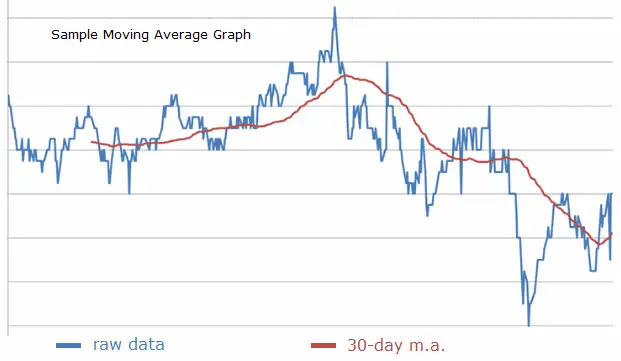# Moving Average Calculator

Given a list of sequential data, you can construct the n-point moving average (or rolling average) by finding the average of each set of n consecutive points. For example, if you have the ordered data set

10, 11, 11, 15, 13, 14, 12, 10, 11,

the 4-point moving average is

11.75, 12.5, 13.25, 13.5, 12.25, 11.75

Moving averages are used to "smooth" sequential data; they make sharp peaks and dips less pronounced because each raw data point is given only a fractional weight in the moving average. The larger the value of n, the smoother the graph of the moving average compared to the graph of the original data. Stock analysts often look at moving averages of stock price data to predict trends and see patterns more clearly. You can use the calculator below to find a moving average of a data set.

 Enter comma-separated data here. DO NOT end list with a comma. Number of Consecutive Points to Average: 2 3 4 5 6 7 8 9 10 11 12 13 14 15 20 21 25 28 30 35 40 50 60 100 Moving average will be output here.#### Number of Terms in a Simple n-Point Moving Average

If the number of terms in the original set is d and the number of terms used in each average is n, then the number of terms in the moving average sequence will be

d - n + 1.

For example, if you have a sequence of 90 stock prices and take the 14-day rolling average of the prices, the rolling average sequence will have 90 - 14 + 1 = 77 points.

This calculator computes moving averages where all terms are weighted equally. You can also create weighted moving averages in which some terms are given greater weight than others. For example, giving more weight to more recent data, or creating a centrally weighted mean where the middle terms are counted more. See the weighted moving averages article and calculator for more information. Along with moving arithmetic averages, some analysts also look at the moving median of ordered data since the median is unaffected by strange outliers.